Open in App
Not now

# Drop rows from the dataframe based on certain condition applied on a column

• Difficulty Level : Easy
• Last Updated : 20 Sep, 2022

In this post, we are going to discuss several approaches on how to drop rows from the Dataframe based on certain conditions applied to a column. Retain all those rows for which the applied condition on the given column evaluates to True.

We have already discussed earlier how to drop rows or columns based on their labels. However, in this post we are going to discuss several approaches on how to drop rows from the dataframe based on certain condition applied on a column. Retain all those rows for which the applied condition on the given column evaluates to True.

## Creating Dataframe to drop rows

In this Dataframe, currently, we are having 458 rows and 9 columns.

## Python3

 `# importing pandas as pd``import` `pandas as pd` `# Read the csv file and construct the``# dataframe``df ``=` `pd.read_csv(``'nba.csv'``)` `# Visualize the dataframe``print``(df.head(``15``)` `# Print the shape of the dataframe``print``(df.shape)`

Output: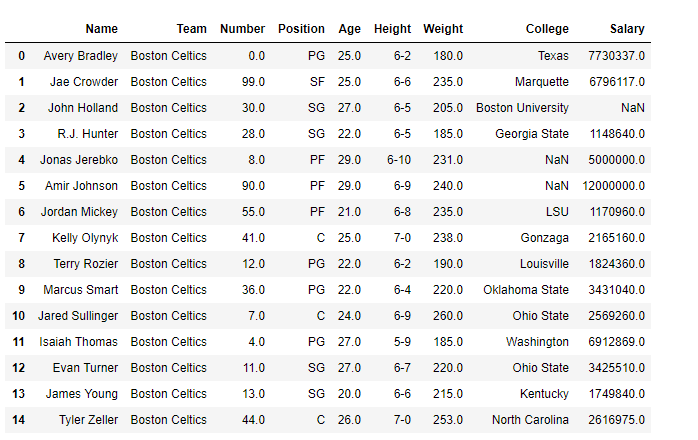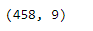## Delete rows based on the condition of a column

We will use vectorization to filter out such rows from the dataset which satisfy the applied condition. Let’s use the vectorization operation to filter out all those rows which satisfy the given condition.

## Python3

 `# Filter all rows for which the player's``# age is greater than or equal to 25``df_filtered ``=` `df[df[``'Age'``] >``=` `25``]` `# Print the new dataframe``print``(df_filtered.head(``15``)` `# Print the shape of the dataframe``print``(df_filtered.shape)`

Output:

As we can see in the output, the returned Dataframe only contains those players whose age is greater than or equal to 25 years.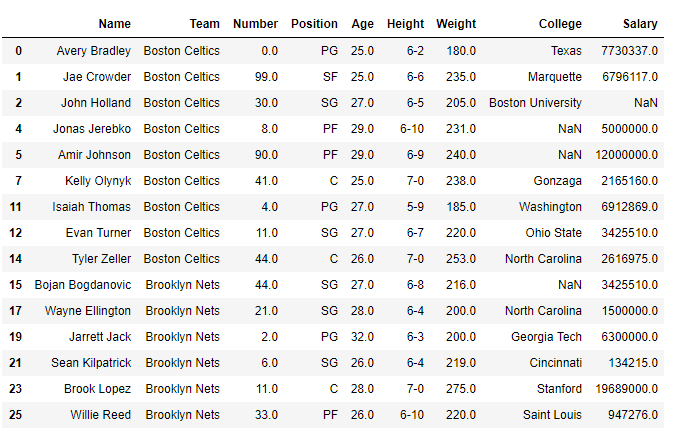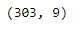## Delete rows based on multiple conditions on a column

As we can see in the output, the returned Dataframe only contains those players whose age is not between 20 to 25 age using df.drop()

## Python3

 `# delete all rows with column 'Age' has value 30 to 40``indexAge ``=` `df[ (df[``'Age'``] >``=` `20``) & (df[``'Age'``] <``=` `25``) ].index``df.drop(indexAge , inplace``=``True``)``df.head(``15``)`

Output: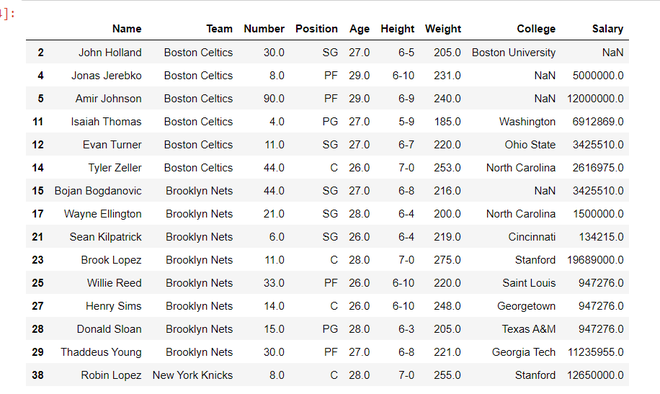## Delete rows based on multiple conditions on different columns

Here, we drop all the rows whose names and Positions are associated with ‘John Holland‘ or ‘SG’ using df.drop().

## Python3

 `# delete all rows with column 'Age' has value 30 to 40``indexAge ``=` `df[ (df[``'Name'``] ``=``=` `'John Holland'``) | (df[``'Position'``] ``=``=` `'SG'``) ].index``df.drop(indexAge , inplace``=``True``)``df.head(``15``)`

Output: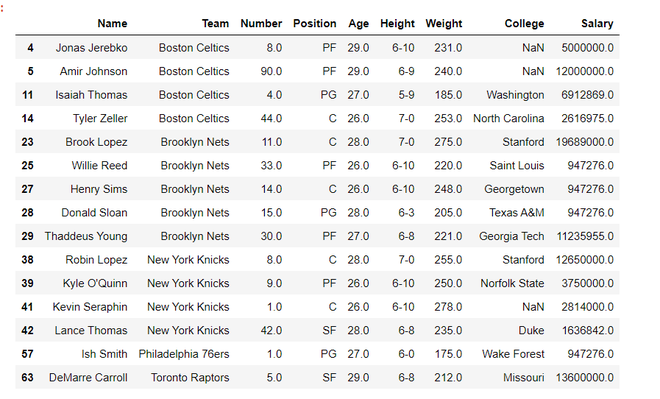RECOMMENDED ARTICLES – how to drop rows or columns based on their labels

My Personal Notes arrow_drop_up